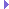Triangulator3 Class Reference

This is an extension of Triangulator to handle polygons with three- dimensional points. More...

Inheritance diagram for Triangulator3:## Public Member Functions

__init__ ()

__init__ (const Triangulator3)

int addVertex (const LPoint3d point)
Adds a new vertex to the vertex pool. More...

int addVertex (double x, double y, double z)
Adds a new vertex to the vertex pool. More...

clear ()
Removes all vertices and polygon specifications from the Triangulator, and prepares it to start over. More...

int getNumVertices ()
Returns the number of vertices in the pool. More...

const LPlaned getPlane ()
Returns the plane of the polygon. More...

const LPoint3d getVertex (int n)
Returns the nth vertex. More...

list getVertices ()

triangulate ()
Does the work of triangulating the specified polygon. More...Public Member Functions inherited from Triangulator
__init__ ()

__init__ (const Triangulator)

addHoleVertex (int index)
Adds the next consecutive vertex of the current hole. More...

addPolygonVertex (int index)
Adds the next consecutive vertex of the polygon. More...

int addVertex (const LPoint2d point)
Adds a new vertex to the vertex pool. More...

int addVertex (double x, double y)
Adds a new vertex to the vertex pool. More...

beginHole ()
Finishes the previous hole, if any, and prepares to add a new hole. More...

clear ()
Removes all vertices and polygon specifications from the Triangulator, and prepares it to start over. More...

clearPolygon ()
Removes the current polygon definition (and its set of holes), but does not clear the vertex pool. More...

int getNumTriangles ()
Returns the number of triangles generated by the previous call to triangulate(). More...

int getNumVertices ()
Returns the number of vertices in the pool. More...

int getTriangleV0 (int n)
Returns vertex 0 of the nth triangle generated by the previous call to triangulate(). More...

int getTriangleV1 (int n)
Returns vertex 1 of the nth triangle generated by the previous call to triangulate(). More...

int getTriangleV2 (int n)
Returns vertex 2 of the nth triangle generated by the previous call to triangulate(). More...

const LPoint2d getVertex (int n)
Returns the nth vertex. More...

list getVertices ()

bool isLeftWinding ()
Returns true if the polygon vertices are listed in counterclockwise order, or false if they appear to be listed in clockwise order. More...

triangulate ()
Does the work of triangulating the specified polygon. More...

## Public Attributes

const LPlaned plane
Returns the plane of the polygon. More...

const LPoint3d vertices []Public Attributes inherited from Triangulator
const LPoint2d vertices []

## Detailed Description

This is an extension of Triangulator to handle polygons with three- dimensional points.

It assumes all of the points lie in a single plane, and internally projects the supplied points into 2-D for passing to the underlying Triangulator object.

## ◆ __init__() [1/2]

 __init__ ( )

## ◆ __init__() [2/2]

 __init__ ( const Triangulator3 )

## ◆ addVertex() [1/2]

 int addVertex ( const LPoint3d point )

Adds a new vertex to the vertex pool.

Returns the vertex index number.

## ◆ addVertex() [2/2]

 int addVertex ( double x, double y, double z )

Adds a new vertex to the vertex pool.

Returns the vertex index number.

## ◆ clear()

 clear ( )

Removes all vertices and polygon specifications from the Triangulator, and prepares it to start over.

## ◆ getNumVertices()

 int getNumVertices ( )

Returns the number of vertices in the pool.

Note that the Triangulator might append new vertices, in addition to those added by the user, if any of the polygon is self-intersecting, or if any of the holes intersect some part of the polygon edges.

## ◆ getPlane()

 const LPlaned getPlane ( )

Returns the plane of the polygon.

This is only available after calling triangulate().

## ◆ getVertex()

 const LPoint3d getVertex ( int n )

Returns the nth vertex.

## ◆ getVertices()

 list getVertices ( )

## ◆ triangulate()

 triangulate ( )

Does the work of triangulating the specified polygon.

After this call, you may retrieve the new triangles one at a time by iterating through get_triangle_v0/1/2().

## ◆ plane

 const LPlaned plane

Returns the plane of the polygon.

This is only available after calling triangulate().

## ◆ vertices

 const LPoint3d vertices[]